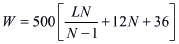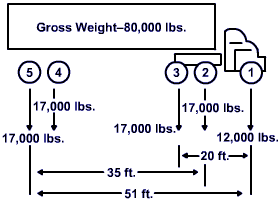ft.
in.
Axles

The Bridge Formula Weights Calculator provides a convenient way to determine the maximum allowable weight that any set of axles on a motor vehicle may carry on the Interstate highway system.

Congress enacted the Bridge Formula in 1975 to limit the weight-to-length ratio of a vehicle crossing a bridge. This is accomplished either by spreading weight over additional axles or by increasing the distance between axles. Compliance with the Bridge Formula weight limits is determined by using the following formula:• W = the overall gross weight on any group of two or more consecutive axles to the nearest 500 pounds.
• L = the distance in feet between the outer axles of any group of two or more consecutive axles.
• N = the number of axles in the group under consideration.Federal law states that two or more consecutive axles may not exceed the weight computed by the Bridge Formula even though single axles, tandem axles, and gross vehicle weights are within legal limits. As a result, the axle group that includes the entire truck—sometimes called the "outer bridge" group—must comply with the Bridge Formula. Interior combinations of axles, such as the "tractor bridge" (axles 1, 2, and 3) and "trailer bridge" (axles 2, 3, 4, and 5) must also comply with weights computed by the Bridge Formula (see figure).

The maximum gross vehicle weight allowed by 23 USC 127 is 80,000 lbs., except where a lower gross vehicle weight is dictated by the Bridge Formula. For further details see 23 CFR Part 658.

All information provided by the Office of Operations, Federal Highway Administration, U.S. Department of Transportation